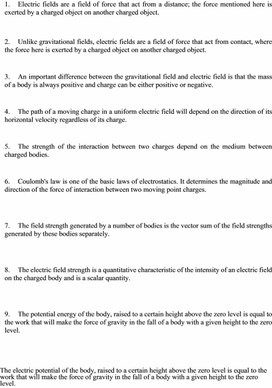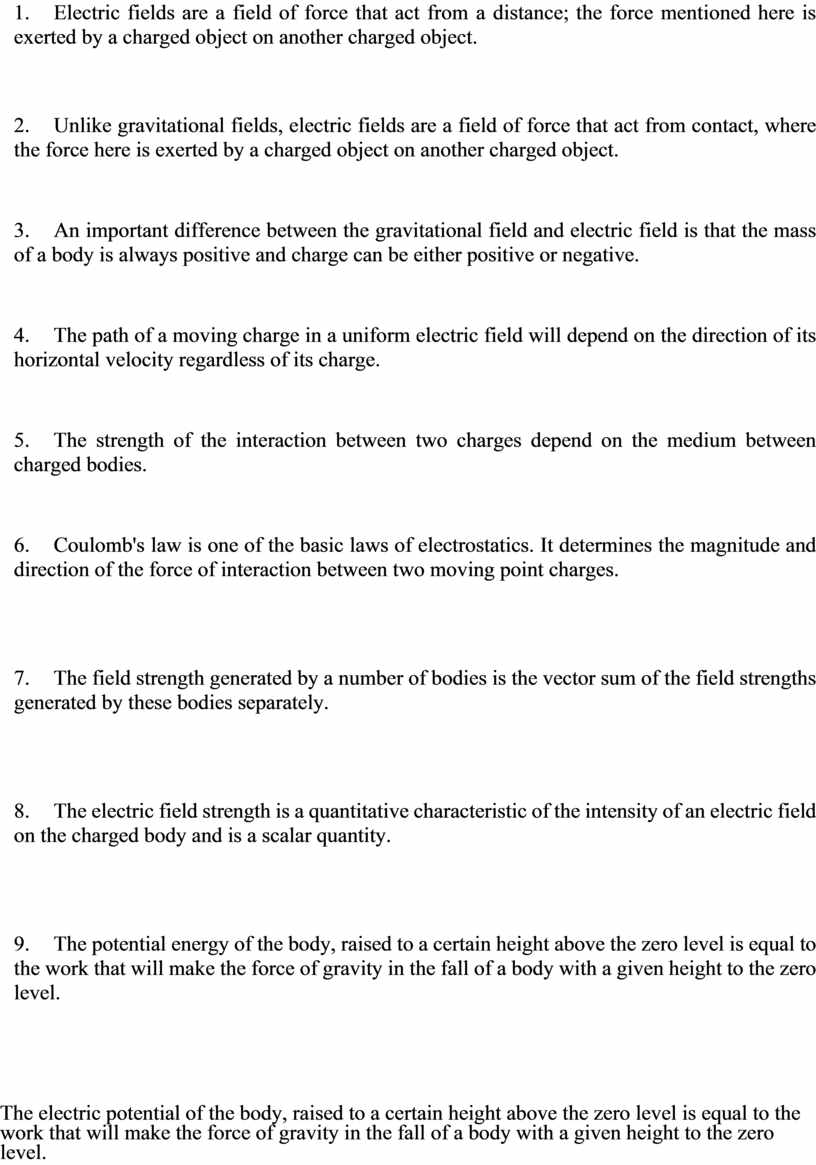Истина или ложь
Оценка 4.6

# Истина или ложь

Оценка 4.6
doc
07.05.2020Истина или ложь.doc

1.           Electric fields are a field of force that act from a distance; the force mentioned here is exerted by a charged object on another charged object.

2.           Unlike gravitational fields, electric fields are a field of force that act from contact, where the force here is exerted by a charged object on another charged object.

3.           An important difference between the gravitational field and electric field is that the mass of a body is always positive and charge can be either positive or negative.

4.           The path of a moving charge in a uniform electric field will depend on the direction of its horizontal velocity regardless of its charge.

5.           The strength of the interaction between two charges depend on the medium between charged bodies.

6.           Coulomb's law is one of the basic laws of electrostatics. It determines the magnitude and direction of the force of interaction between two moving point charges.

7.           The field strength generated by a number of bodies is the vector sum of the field strengths generated by these bodies separately.

8.           The electric field strength is a quantitative characteristic of the intensity of an electric field on the charged body and is a scalar quantity.

9.           The potential energy of the body, raised to a certain height above the zero level is equal to the work that will make the force of gravity in the fall of a body with a given height to the zero level.

10.      The electric potential of the body, raised to a certain height above the zero level is equal to the work that will make the force of gravity in the fall of a body with a given height to the zero level.

11.

## Electric fields are a field of force that act from a distance; the force mentioned here is exerted by a charged object on another charged…Скачать файл